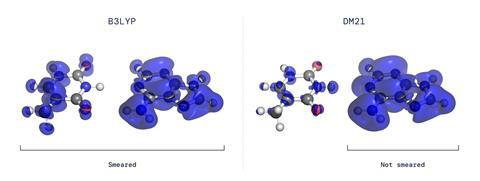A neural network constructed by researchers at Google’s artificial intelligence arm DeepMind has produced a refined density functional theory algorithm. The algorithm, which considers ‘fractional electronic character’ overlooked in previous DFT algorithms, is able to avoid some of the pitfalls that often lead these algorithms to give highly inaccurate results.

The Schrödinger equation cannot be solved analytically for any atomic system more complex than the hydrogen atom because electron–electron repulsion means the variables involved increase dramatically. This means that to create realistic approximations of molecular systems, researchers have to turn to computational methods. For some small molecules, it is feasible to find approximate numerical solutions to the equation for each electron and estimate the shape of each molecular orbital from the resulting wavefunctions. However, the computational demands are huge and grow steeply, as every additional electron repels every other. ‘The question is how do you go beyond this area?’ says DeepMind’s Aron Cohen.

In the 1960s, Pierre Hohenberg and Walter Kohn showed that, in principle, one could map a molecule’s electron density precisely to its energy. As a molecule would tend to adopt its lowest energy state, chemists could theoretically predict the state of a molecule without knowing the state of any single electron. Kohn later shared the 1998 Nobel prize in chemistry for his work, which gave birth to DFT. The work does not, however, reveal how to compute the electron density, and researchers have worked to find better ‘density functionals’ to do this ever since.

Theoretical chemists have worked out an ever-growing list of constraints that accurate density functionals should satisfy. Two of these, which concern the delocalisation of electronic spin and charge between atoms in a molecule, are highly problematic. ‘For example, if you have H2+ in this system on average you will have half of the electron on one hydrogen and half of the electron on the other side,’ explains Cohen’s colleague James Kirkpatrick. ‘Most functionals predict that, even at infinite distance, those two atoms are bonded – this is a large error in DFT caused by the fact that functionals get confused by the fact that it’s just one half of a whole electron.’

The researchers created a self-learning neural network and trained it on fictitious systems. ‘If one thinks of an atom, one can also think of the electron density if one removes an electron or half an electron,’ explains Cohen. Through a process of feedback and self-correction, the neural network learned to predict the electron density around small molecules.